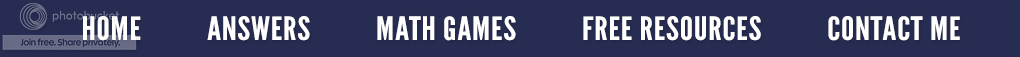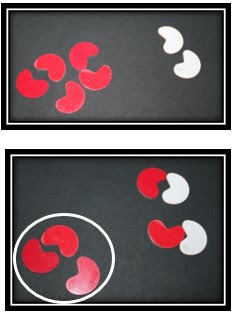### Using Two-Sided Colored Beans to Add and Subtract Positive and Negative Numbers

When it comes to adding and subtracting positive and negative numbers, many students have great difficulty. In reality, it is a very confusing and abstract idea; so, it is important to give the students a concrete visual to assist them in seeing the solution. This idea is based on the Conceptual Development Model which is important to use when introducing new math concepts. (See the August 14, 2019 post for more details about this learning model.) As a result, when teaching the concept of adding and subtracting positive and negative numbers, what would fall into each category?

When using the two-sided colored beans, the concrete stage of the Model would be where two-sided colored beans are used as an actual manipulative that can be moved around or manipulated by the students. There are a few rules to remember when using the beans.
1. The RED beans represent negative numbers.
2. The WHITE beans represent positive numbers.
3. One RED bean can eliminate one WHITE bean, and one WHITE bean can cancel out one RED bean.
4. All problems must be rewritten so that there is only one sign (+ or -) in front of each number.
Sample Problem1) The student is given the problem - 5 + 2.

2) Since -5 is negative, the student gets out five red beans, and then two white beans because the 2 is positive.

3) Since some of the beans are red and two are white, the student must match one red bean with one white bean. (I tell my students that this is barbaric because the red beans eat the white beans. They love it!)

4) Because three red beans have no partner (they're left over) the answer to – 5 + 2 = - 3. (See example above.)

After mastering the concrete stage of the Conceptual Development Model, the students would move on to the pictorial stage. Sketching a picture of the beans would be considered pictorial. Have students draw circles to represent the beans, leaving the circles that denote positive numbers white and coloring the circles that represent negative numbers red.

As an example, let’s do the problem 3 - +5. First, rewrite the problem as 3 - 5. Now draw three white beans. Draw five more beans and color them red to represent -5. Match one white bean to one red bean. Two red beans are left over; therefore, the answer to 3 - +5 is -2.

3 - +5 = 3 – 5 = -2

When students understand the pictorial stage, then abstract problems such as the ones in textbooks can be presented. (Notice, the textbook is the last place we go for an introduction.) I have found that most of my remedial college students move straight from the concrete stage (beans) to the abstract stage without any problem. Many put away the beans after two or three lessons. What works best for your students as they master this algebraic concept is something you will have to determine.Only \$5.25
If you would like a resource that gradually goes through these lessons, you can purchase it on Teachers Pay Teachers. It introduces the algebraic concept of adding and subtracting positive and negative numbers and contains several integrated hands-on activities. They include short math lessons with step-by-step instructions on how to use the beans, visual aids and illustrations, four separate and different practice student worksheets with complete answers in addition to detailed explanations for the instructor.

#### 1 comment:

Kathie @Tried and True Teaching Tools said...

Vicky, I had never thought of using the 2-sided beans to teach negative numbers; I love this! Funny story: when my daughter was in kindergarten, her teacher had them use these beans to teach addition. My daughter hated to color (and still hates it; she's in high school now!) so "somehow" all her tosses of the beans resulted in 5 (white) + 0 (red) = 5. Her teacher commented on the (highly unlikely) probability of getting all white beans on every single toss & made her redo the lesson; LOL